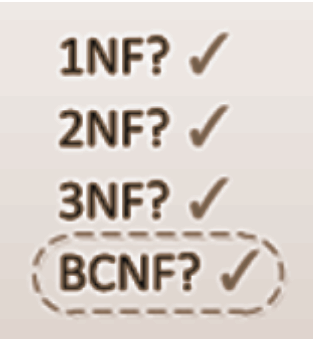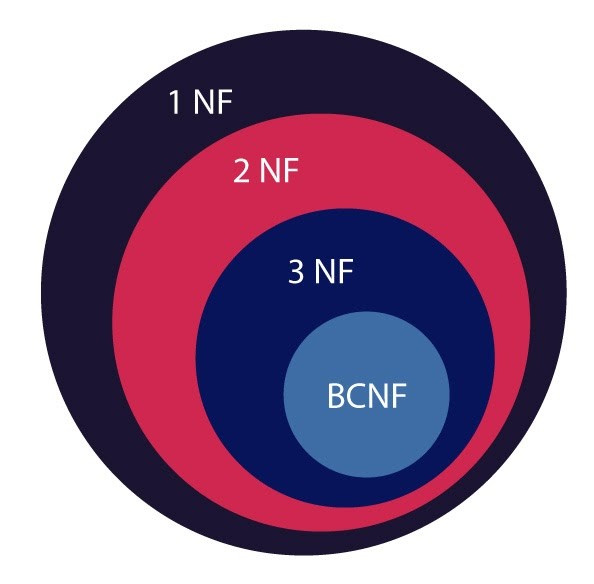# Highest Normal Form of a Relation

## Introduction

Normalization refers to organizing data within a database by creating tables and establishing relationships between them to protect the data and make the database more efficient and flexible. Usually, we consider every table in a relational database to be in the first normal form, which says the tuples must be atomic, which means there must be no multiple values in a single row.

The table must be free of any partial dependencies to be in the second normal form. The table must not have any transitive dependency for the third normal form. Finally, to be in BCNF, the determinant in the given dependency must be a super key.This article will learn how to find the highest normal form of a given relation after revising all the terms related to 2NF, 3NF, and BCNF.

## Important terms in Normalization

Attribute: An attribute in a table describes characteristic defining items under a single category in the cells of a column.

Superkey: A super key is an attribute or set that uniquely identifies data items in a table. Superkey attributes can contain null values too, which is not the case for a primary key.

Candidate key: We could say that the candidate key is a super key to records in the table. A candidate key can either be an attribute (column) or a group of attributes that uniquely identify every data item, i.e., all individual records in a relation or a table. A Candidate Key is a Super Key whose no proper subset is a super key.

Non-prime attributes: All other columns in a relation that do not occur in any candidate key are non-prime attributes.

Prime-attributes: Any candidate key column will make a prime attribute.

Dependency: In a relation, dependency is a constraint defining a relationship between two or more attributes. Dependency occurs when data stored in the same table can be used to determine some other information stored in the table uniquely. There are various dependencies in relational databases, including Functional Dependency, Fully-functionally Dependency, transitional dependency, and Partial Dependency.

Functional dependency: It is a constraint specifying the relationship between two attributes where one attribute helps determine the value of the other. Functional dependency is denoted as X → Y. Here, X is a set of attributes that can help determine the value of Y.

Determinant: The set of attributes to the left of the arrow that helps determine the value of the other attributes is called the determinant. In X→Y, X is the determinant.

Dependent: The attribute to the right of the arrow, whose value is determined by the determinant, is called the dependent. In X→Y, Y is the dependent.

Transitive functional dependency: When a dependent is indirectly dependent on the determinant, it is a transitive functional dependency. This means that if a → b and b → c, then a → c.

## Identifying the Highest Normal Form

Since BCNF is the highest normal form, we are aware that it has the strictest Normalization. This means that any table in BCNF also follows all the conditions for 3NF, 2NF and 1NF(all relations are at least 1NF). The diagram below depicts the same concept. For instance, a relation in BCNF will definitely be in 2NF, but a relation in 2NF may or may not be in BCNF.Therefore, if a table is in BCNF, we can be sure that it is also in third, second and first normal forms. If BCNF fails, we check it for 3NF, otherwise 2NF. Using this bottom-up approach while checking for the highest normal form will save us a lot of time.

## Conditions for all Normal Forms

Before we start checking for any normal form conditions, we must first identify and write down the dependencies in the table. Then, we can proceed to check the relations from the highest to the lowest normal form using these methods:

BCNF: For every dependency from x→y, then x must be a super key irrespective of y being a prime or a non-prime attribute.

3NF: There must not be any partial or transitive dependency. Both of these conditions can be checked together using this method: We know that if x is a super key or a candidate key, the relation could be in BCNF. However, if x is not the superkey, there is a possibility that it is still in 3NF. For x→y, if y is a prime attribute, there would be no partial or transitive dependency (because y is non-prime in both partial and transitive dependency).

Therefore, to check for 3NF for a relation with dependency x→y, we must first check if x is a superkey. If it does not work, we must check if y is a prime attribute, proving the relation in 3NF.

2NF: If the test for the third normal form fails, we will check if, in x→y, y is non-prime, and x is a candidate key, i.e., a prime attribute. If y depends on the part of the candidate key x (partially), then the table has a partial dependency, and the table would not be in 2NF. However, if there is no partial dependency, the relation will be in the second normal form.

## Example questions

1. For a relation R with attributes (A, B, C, D, E, F, G, H) with the following functional dependencies:

Where AB is the candidate key, find the highest normal form.

Solution: We will start with checking for BCNF. Is AB a superkey? Since AB is a candidate key, it is also a superkey. Therefore, BCNF applies to the first dependency. Now for the second dependency, is A a superkey? No, meaning this dependency would not be in BCNF. (for x→y to have BCNF, x must be a superkey (or candidate key).

Now, if any dependency in a table is not in BCNF, we know that the table isn’t in BCNF. Therefore, we will check it for 3NF, i.e., for x→y, either x must be a superkey or y is a prime attribute. Picking it up from where we left checking for BCNF, A is not a superkey. DE is not a prime attribute either because with AB being the superkey, only A and B are prime attributes. This means the table is not even in 3NF.

Checking for 2NF, we will check for partial dependency. First, we have already deduced that D and E are non-prime; therefore, DE is a non-prime attribute. Second, we will check if A is the entire candidate key or a part of the candidate key. The candidate key is AB and A is just a part of it. Hence, DE only depends on the part of the candidate key, proving partial dependency in the table. The presence of partial dependency means that the table is not in 2NF either. Therefore, the highest normal form of the relation R is 1NF.

2. For a relation R (A, B, C, D, E, F) with the following functional dependencies:

Find the highest normal form where ABD and BCD are the candidate keys.

Solution: For BCNF, is AB a superkey? No, only ABD and BCD are the candidate keys. BCNF fails in the very first dependency. So we will check it for 3NF. We already know AB isn’t a candidate key or a superkey, but C is a prime attribute since it is a part of BCD (candidate key). Therefore the dependency is in the third normal form.

For the second dependency, DC is not a part of any candidate key, but AE must be a prime attribute. We can see that A is a prime attribute because it is part of a candidate key, but  E isn’t. Hence this dependency is not in the third normal form either.

For the first dependency, C is not a non-prime attribute because it is a part of a candidate key. For the second dependency DC→AE, we can split it into DC→A and DC→E. E is a non-prime attribute depending on the part of the candidate key, which is a case of partial dependency. Again, the table is not even in the second normal form.

The highest normal form of this example is 1NF.

3. For a relation R (V, W, X, Y, Z), VW, WY, WX are the candidate keys. These are the following functional dependencies:

Find the highest normal form of the relation.

Solution: For FD: VW->XY, VW is a super key; hence it is in BCNF. However, Y is not a candidate key, so the second dependency is not BCNF.

Checking for 3NF, VW is a candidate key. Y is not a candidate key but V is a prime attribute since it is a part of the candidate key (VW).

For the last dependency, we don’t need to split WX→YZ into WX→Y and WX→Z. This is because WX is already a candidate key. Therefore, this relation is in 3NF.

1. What is the highest normal form in a relational database?
Boyce-Codd Normal Form is an advanced version of the third normal form in database normalization. It is the strictest, highest and most efficient form of Normalization since it eliminates the condition that allowed the right side of the functional dependency to be a prime attribute in 3NF. Thus, BCNF is stronger than 3NF because every left side in a functionally dependent relation must be a superkey.

2. Why do we check for the highest normal form using the bottom-up approach?
A table will only be in BCNF if it fulfils all the conditions for 2NF and 3NF first. Therefore, any table in BCNF will automatically be in 3NF, 2NF and 1NF(all relations are at least 1NF) while following all the conditions for them. Hence, it will only make sense to check from the top to the bottom as it will save us time while solving questions.

3. What are functional dependencies?
Functional dependencies are essentially the relationship between attributes in a table. They usually exist between the primary key and non-key attributes. If a functional dependency is denoted as X → Y, X is a set of attributes that can help determine the value of Y.
A functional dependency is hence a constraint specifying the relationship between two attributes where one attribute helps determine the value of the other.

## Key takeaways

In this article, we learned about the important terms about Normalization, the conditions that help identify various normal forms and the technique to find the highest normal form. We also used example questions and their detailed solutions to understand the concept better and learn to use our knowledge of the same.

Check out our top 100 SQL problems at coding ninjas which are useful for a huge range of product and service-based companies. You can find a lot of interesting modules on Database Management Systems at Coding Ninjas. Visit CodeStudio to try and solve more problems for practice. Share this blog with your friends if you found it helpful! Until then, All the best for your future endeavours, and Keep Coding.